# Project Management Formulas

There are a number of project management formulas to perform quantitative analysis, but from pmp exam point of view there are a few important ones. Key areas such as Earned Value Analysis, Critical Path Analysis, and Procurement Management require use of Quantitative skills. Hence, this post enumerates important mathematical formulas used in the field of Project Management.

## Project Management Formulas for PMP Certification Examination

### Project Selection Methods

#### Net Present Value (NPV) Project Management Formulas

Present Value (PV) = Future Value (FV) / (1+k)N• Where k = discounting factor / interest rate and N = Number of time periods

NPV = [Sum of present value of all future cash inflows] – [Cash outflow]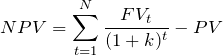While evaluating two or more projects select the project with greater NPV

#### Internal Rate of Return (IRR)

• IRR is the discount rate at which NPV = 0

#### Benefit Cost Ratio (BCR) / Cost Benefit Analysis (CBA)

Ratio of sum of present value of all cash inflows to project cash outflow.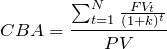#### Return on Investment (ROI)

ROI = Average Income / Average Investment

#### Payback Period

Payback Period (PB) = Initial Investment / Annual Cash Inflow

### Project Selection Acceptance Criteria Summary

Project Selection MethodProject Selection CriteriaProject Selection Decision
Net Present Value (NPV)if NPV is greater than zeroAccept
if NPV is less than zeroReject
Internal Rate of Return (IRR)Greater than thresholdAccept
Less than thresholdReject
Cost Benefit Analysis (CBA)if CBA is greater than oneAccept
if CBA is less than oneReject
Return on Investment (ROI)Greater than thresholdAccept
Less than thresholdReject
Payback periodLess than thresholdAccept
Greater than thresholdReject

### Project Management Formulas for PERT Analysis

Following are the project management formulas for Program Evaluation and Review Technique (PERT)

#### Three Point Estimates (Beta Distribution)

• Activity Duration / Cost Estimates = (P + 4M +O) / 6Where P = Pessimistic Value; M = Most Likely Value; O = Optimistic Value

• Standard Deviation (σ)= (P – O) / 6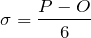• Variance = (σ)2 = (P – O)2 / 36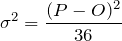#### Three Point Estimates (Triangular Distribution)

• Activity Duration / Cost Estimates = (P + M + O) / 3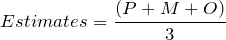Also read: Program Evaluation And Review Technique

### Critical Path Analysis Formulas

#### Early Start (ES)

Project management formulas for Early Start (ES) if the convention that project starts on day one is adopted.

Early Start (ES) =

• 1 for the first activity
• ES of the next node is the earliest finish time of the immediately preceding activity plus 1 = EF + 1

For activities with more than one preceding activity ES is latest of the earliest finish times of the preceding activities.

#### Early Finish (EF)

Project management formula for Early Finish (EF) if the convention that project starts on day one is adopted.

• Early Finish (EF) = ES + Activity Duration (D) – 1

#### Late Finish (LF)

Project management formula for Late Finish (LF) if the convention that project starts on day one is adopted.

Late Finish (LF) =

• On Critical Path LF of the last activity is equal to the EF of that activity
• The latest start time of the activity of previous node minus 1 = LS -1

For activities with more than one previous node, LF is the earliest of the latest start times of those activities.

#### Late Start (LS)

Project management formulas for Late Finish (LS) if the convention that project starts on day one is adopted.

• Late Start (LS) = LF – Activity Duration (D) + 1

#### Project Management Formulas for Critical Path Analysis

• For Critical Path ES = LS and EF = LF
• For Critical Path Total Float = 0

#### Total / Free Float

Project management formulas to calculate total float / free float.

• Total Float = LS – ES or LF – EF
• Free Float = (ES)S – (EF)C – 1 [When Start = 1] Where S = Successor Activity and C = Current ActivityCritical Path Analysis Forward and Backward Pass Calculations

#### Also Read Post Related to Critical Path Analysis

Read the following post to know about Critical Path Analysis formulas if the convention that project starts on day Zero is selected.

### Cost to Crash

The following equation gives project management formula to calculate cost to crash.

• Cost to crash per period = (CC – NC) / (NT – CT)
• CC = The activity cost associated with the crash time
• NC = The activity cost associated with the normal time
• NT = The time necessary to complete the activity under normal conditions
• CT = The shortest possible time necessary to complete an activity

### Cost Estimates

The following are the two types of cost estimates.

• Rough Order of Magnitude (ROM) Estimates = -25% to +75%
• Definitive Estimates = -5% to +10%

### Project Budget

The following paragraph lists project management formulas to compute project budget.

• Work Package Cost Estimates = Activity Cost Estimates + Activity Contingency Reserve
• Control Accounts = Work Package Cost Estimates + Contingency Reserve
• Cost Baseline = Summation of Control Accounts
• Project Budget = Cost Baseline + Management Reserve

### Number of Communication Channels

Project management formulas to calculate total number of communication channels

• Number of communication channels = N(N – 1) / 2 ; Where N = Number of stakeholders

### Project Management Formulas for Expected Monetary Value (EMV)

The following paragraphs lists various formulas in expected monetary value calculations.

• EMV = Probability X Net Path Value
• Net Path Value = Pay offs – Costs along the path
• EMV of opportunities = Positive Value
• EMV of risks = Negative Value

### Project Management Formulas for Procurement Management

The following paragraphs lists various formulas in project procurement management.

• Target Price = Target Cost + Target Fee
• Final Fee = [(Target Cost – Actual Cost)*Sellers ratio] + Target Fee
• Final Price = Actual Cost + Final Fee
• Point of Total Assumption (PTA) = [(Ceiling Price – Target price) / Buyer’s Ratio] + Target Cost

## Earned Value Analysis Project Management Formulas

### Earned Value Measurement

#### Fixed Formula

• 25 / 75 Method
• 50 / 50 Method
• EV = (% ) X Work package BAC

#### Percent Complete

• EV = % Work Complete Estimate X Work Package BAC

### Project Management Formulas Earned Value Variance Analysis

The following table enumerates the list of earned value variances formulas in project management.

Earned Value Analysis IndicatorEarned Value Analysis FormulaEarned Value Analysis CriteriaEarned Value Analysis Interpretation
Schedule Variance (SV)SV = EV - PVPositive Value SV > 0Ahead of schedule
Equal to Zero SV = 0On schedule
Negative Value SV < 0Behind Schedule
Cost Variance (CV)CV = EV – ACPositive Value CV > 0Under planned cost
Equal To Zero CV = 0On planned cost
Negative Value CV < 0Over planned cost
Variance at Completion (VAC)VAC = BAC – EACPositive VAC > 0Under planned cost
Equal To Zero VAC = 0On planned cost
Negative VAC < 0Over planned cost

Also read: Variance Analysis In Project Management

### Project Management Formulas Earned Value Performance Indices

The other name for earned value analysis performance indices is efficiency indicators. The following table enumerates the list of formulas for earned value analysis performance indicators.

Earned Value Analysis IndicatorEarned Value Analysis FormulaEarned Value Analysis CriteriaEarned Value Analysis Interpretation
Schedule performance Index (SPI)SPI = EV / PVGreater than 1 SPI > 1Ahead of schedule
Equal to One SPI = 1On schedule
Less than one SPI < 1Behind Schedule
Cost Performance Index (CPI) CPI = EV / ACGreater than 1 CPI > 1Under planned cost
Equal to One CPI = 1On planned cost
Less than one CPI < 1Over planned cost

Also read: Earned Value Analysis Project Management

### Earned Value Analysis Forecasting Project Performance

#### Estimate To Complete (ETC)

Project management formulas to calculate Estimate to complete (ETC) to complete the balance work.

• Method 1 to calculate ETC
• ETC = Re-estimate manually
• Method 2 using mathematical equation
• ETC = (BAC-EV)/CPI
• Method 3 if EAC is valid then;
• ETC = EAC – AC

#### Estimate At Completion (EAC)

Project management formulas to compute Estimate at Completion (EAC) for the balance work.

• If future work will be accomplished at the planned rate (EAC at budgeted rate)
• EAC = AC + BAC – EV
• If the CPI is expected to be the same (EAC at current CPI)
• EAC = BAC/CPI
• Considering impact of both CPI and SPI
• EAC = AC + [(BAC – EV) / (CPI x SPI)]
• If the initial plan is no longer valid then
• EAC = AC + Bottom-up ETC

#### To Complete Performance Index (TCPI)

Project management formulas to calculate TCPI

• TCPI = (BAC – EV) / (BAC-AC)
• TCPI = (BAC-EV) / (EAC-AC)
• Work Remaining = BAC – EV
• Funds Remaining = BAC – AC
• Also, Funds Remaining = EAC – AC
• Greater than 1.0 = Harder to complete
• Exactly 1.0 = Same to complete
• Less than 1.0 = Easier to complete

### Earned Value Management Quiz

Take the Earned Value Management Quiz

## Statistics

### Mean

• Mean (µ) = ∑ X / N = (X1 + X2 + X3 + . . . . XN) / N

### Variance

• Variance (σ2) = ∑ (X – µ)2/ N

### Standard Deviation

Standard deviation (σ) is square root of variance.

If a set of data is normally distributed then 68% of the data values fall within one standard deviation (µ ± 1σ) of the mean, 95% are within (µ  ± 2σ ) and 99.7% with in (µ ± 3σ).

Also read: PMP Certification Exam Changes 2019

## Summary

I hope I have compiled as many formulas as possible. In case I have missed any important formula please do let me know.

## 20 thoughts on “Project Management Formulas”

1.aconex oracle says:

Using the objectivity of time and costs, you can assign small yet measurable chunks of work to your teams and pick up the pace. Project managers are notified in case of deviation to take necessary proactive steps to bring the project back on track.

2.likhitha says:

I wanted to leave a little comment to support you and wish you a good continuation. Wishing you the best of luck for all your blogging efforts.

1.Atul Gaur PMP says:

Thank you

2.Mangesh C says:

1.Atul Gaur PMP says: